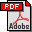A fast and space-economical algorithm for length-limited coding Authors: Jyrki Katajainen, Alistair Moffat, and Andrew Turpin Published in: Proceedings of the 6th Annual International Symposium on Algorithms and Computation, Lecture Notes in Computer Science 1004, Springer-Verlag (1995), 12-21 Full text:PDF (648 kB) DOI: 10.1007/BFb0015404 Copyright: © Springer-Verlag Abstract: The optimal prefix code problem is to determine a vector of integer codeword lengths l = [li  |  i ∈ {1… n}], given an n-vector of symbol frequencies p = [pi  |  i ∈ {1… n}], such that ∑i=1n 2−li ≤ 1, and ∑i=1n li pi is minimised. An extension of the problem is the optimal length-limited prefix code problem, in which the further constraint li ≤ L is imposed, for all i ∈ {1… n} and some integer L ≥ ⌈log2 n⌉. The package-merge algorithm of Larmore and Hirschberg generates length-limited codes in O(nL) time using O(n) words of auxiliary space. In this paper we show how the size of this work space can be reduced to O(L2). This represents a substantial improvement, since for practical purposes L is Θ(log n). The modified algorithm can also be applied to the generation of optimal prefix codes, and it reduces the memory space required for some instances of that problem. BibLATEX: ```@inproceedings{KMT1995bC, author = {Jyrki Katajainen and Alistair Moffat and Andrew Turpin}, title = {A fast and space-economical algorithm for length-limited coding}, booktitle = {Proceedings of the 6th Annual International Symposium on Algorithms and Computation}, series = {Lecture Notes in Computer Science}, volume = {1004}, publisher = {Springer-Verlag}, year = {1995}, pages = {12--21}, } ```